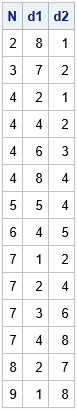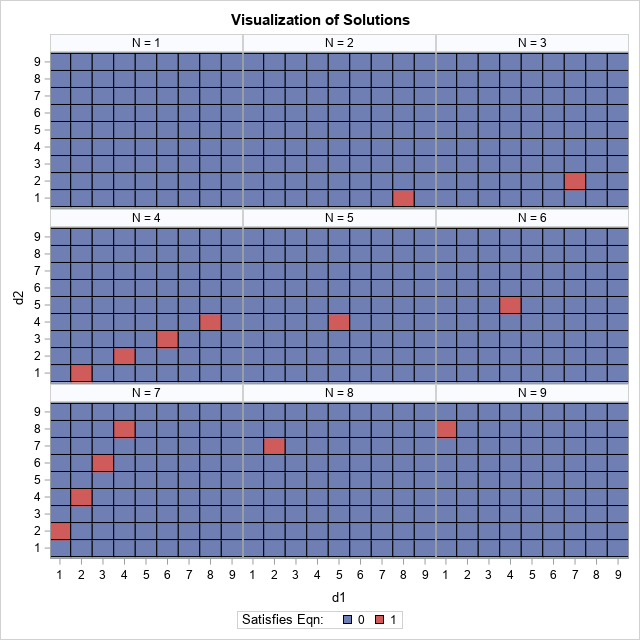I recently discussed introductory programming with a colleague who teaches Python at a university. He told me about the following introductory programming assignment:

Let N be an integer parameter in the range [1, 9]. For each value of N, find all pairs of one-digit positive integers d1 and d2 that satisfy the equation
N*(d2 + d1) = d2d1
where the right-hand side is a two-digit integer. For example, when N=2, the equation has the solution (d1, d2) = (8, 1) because 2*(1+8) = 18.

Most students solve the problem by using a brute-force approach that involves three nested loops that iterate over N, d1, and d2 and output the (d1, d2) pairs that satisfy the equation for each value of N. Notice that all variables are integers in the range [1,9].

This article shows how to solve the problem by using the SAS DATA step. If you want to try to solve the problem yourself without any further hints, stop reading and start programming!

### From words to equations

The ability to translate words to mathematical equations is a skill that students must develop. For this problem, a possible source of confusion is the meaning of the phrase "the two-digit integer d2d1." The students must figure out that the phrase means that d2 is in the "tens" place and d1 in the "ones" place of the two-digit number. In terms of a mathematical equation, the right-hand side is the quantity 10*d2 + d1. If the student can construct the equation, then the rest of the problem is straightforward to program.

### A DATA step solution

The brute-force solution is easy to program because the program iterates of a small number of integers:

• Write a loop that iterates over the possible values of the N parameter.
• For each value of N, write a pair of loops that iterate over all possible (d1, d2) pairs.
• For each (d1, d2) pair, determine if the integers satisfy the equation N*(d2 + d1) = 10*d2 + d1.

The following DATA step implements this strategy:

```data ProgAssignment; do N = 1 to 9; do d1 = 1 to 9; /* "ones" place */ do d2 = 1 to 9; /* tens place */ Test = ( N*(d2 + d1) = 10*d2 + d1 ); /* binary indicator */ output; /* or use IF TEST THEN OUTPUT; */ end; end; end; run;   proc print data=ProgAssignment noobs; where Test=1; var N d1 d2; run;```The programmer needs to determine whether to output all (d1, d2) pairs or just the ones that satisfy the equation. For this implementation, I chose to create a data set of all pairs and to create a binary variable (TEST) that indicates which pairs are solutions. The WHERE clause in the PROC PRINT call prints only the solutions. You can see that most values of N give rise to a unique solution, but for N=4 and N=7, there are multiple solutions. That is because the equation for N=4 is
4*(d2 + d1) = 10*d2 + d1
or
2 d2 = d1
Thus, all even values of d1 are solutions. The case for N=7 is similar.

### Visualize the solutions

One reason that I chose to output all pairs (and not just the solutions) is so that I could create a panel of heat maps that shows the solutions and non-solutions to this problem for each value of the parameter, N. The following call to PROC SGPANEL in SAS creates a visualization of the solutions:

```title "Visualization of Solutions"; proc sgpanel data=ProgAssignment; panelby N / columns=3; heatmapparm x=d1 y=d2 colorgroup=Test / discretex discretey outline; keylegend / title="Satisfies Eqn:"; run;```The panel shows that the equation has no solutions when N=1, multiple solutions when N=4 and N=7, and a unique solution for the remaining values of N. The panel also shows a symmetry in the problem. In practice, you only need to solve the equation for N ≤ 5. For larger values of N, you can switch d1 and d2 and map the problem to one that you have already solved. I leave the details to the reader.

### A vectorized solution

Statistical programmers who use a matrix-vector programming language can solve this problem without writing any loops. This is known as vectorizing the problem. For example, in the SAS IML language, you can use the EXPANDGRID function to generate all (N, d1, d2) triplets. You can then use the LOC function to find all rows that satisfy the equation, as follows:

```proc iml; V = ExpandGrid( 1:9, 1:9, 1:9 ); /* create all combinations of (N,d1,d2) */ N = V[,1]; d1 = V[,2]; d2 = V[,3]; /* for convenience, extract columns */ idx = loc( N#(d2+d1) = 10#d2 + d1 ); /* find all solutions */   Solution = V[idx,]; /* subset of solutions */ print Solution[c={'N' 'd1' 'd2'}];```

The output is identical to the earlier output from PROC PRINT so is not shown.

### A programming challenge

Do you like SAS programming challenges like this? How about this generalization: Let the parameter N ≤ 30 be a positive integer. Find all triplets of one-digit positive integers (d1, d2, d3) that satisfy the equation
N*(d3 + d2 + d1) = d3d2d1
where the right-hand side is a three-digit integer. For example, when N=11, the equation has the solution (d1, d2, d3) = (8, 9, 1) because 11*(1+9+8) = 198. (Hint: There are 41 solutions for N ≤ 30.)

### Summary

This article discusses how to solve an integer programming problem by using a brute-force programming technique that involves nested loops. In a matrix-vector programming language, you can perform the same computations without writing any loops.

ShareDistinguished Researcher in Computational Statistics

Rick Wicklin, PhD, is a distinguished researcher in computational statistics at SAS and is a principal developer of SAS/IML software. His areas of expertise include computational statistics, simulation, statistical graphics, and modern methods in statistical data analysis. Rick is author of the books Statistical Programming with SAS/IML Software and Simulating Data with SAS.

1.Rick,
RobPratt would think this is a piece of cake for SAS/OR , especially for a large N and many d variables.

proc datasets library=work nolist nodetails kill;
quit;
%macro introduce(n=);
%do i=1 %to &n.;
proc optmodel;
var d{1..3} integer >=1 <=9;
con con: &i.*(d+d+d)=100*d+10*d+d ;
solve with clp/findallsolns;
create data want_&i. from [solution]={s in 1.._NSOL_} n=&i. d1=d.sol[s] d2=d.sol[s] d3=d.sol[s];
quit;
%end;
%mend;
ods select none;
%introduce(n=30);
ods select all;
data all;
set want:;
run;
proc print data=all ;run;

•Nice! Thanks for sharing. I originally wrote a paragraph about how to solve this problem by using more sophisticated methods, but I decided to delete it to make the article shorter. When I first saw the problem, I thought I could formulate it as a constrained linear programming problem. However, I realized that there is no objective function, only the constraints. However, as you have pointed out, the CLP solver in SAS/OR enables you to obtain solutions that satisfy the constraint without specifying an objective. I might blog about this problem in the future; I don't think the CLP solver is as well known as it should be.